# ML Aggarwal Class 8 Solutions for ICSE Maths Chapter 2 Exponents and Powers Ex 2.1

## ML Aggarwal Class 8 Solutions for ICSE Maths Chapter 2 Exponents and Powers Ex 2.1

Question 1.
Evaluate:Solution:Question 2.
Simplify: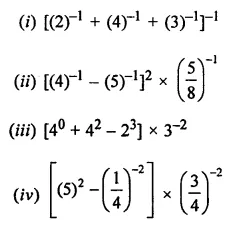Solution: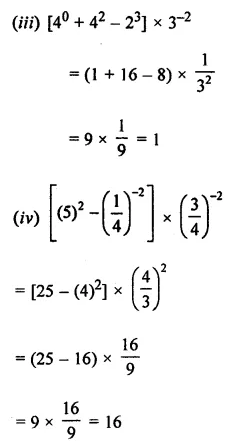Question 3.
Find the multiplicative inverse of the following:Solution: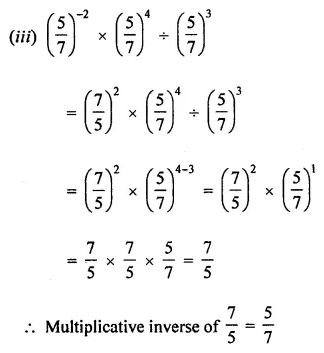Question 4.
(i) Express 16-2 as a power with base 2.
(ii) Express 125-4 as a power with base 5.
Solution:Question 5.
Write the following numbers in expanded form using exponents:
(i) 2789.453
(ii) 3007.805
Solution: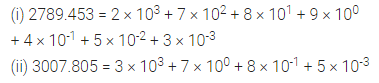Question 6.
Simplify and write in exponential form with positive exponent:Solution: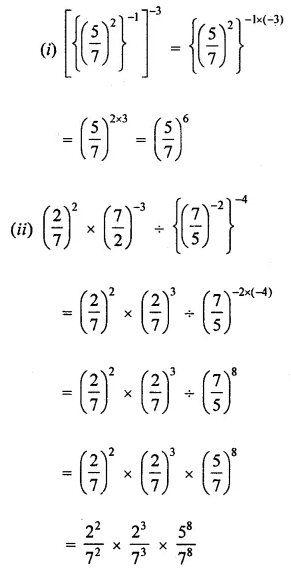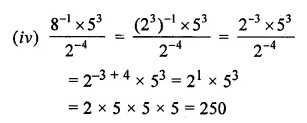Question 7.
Simplify and write the following in exponential form: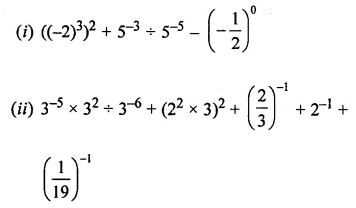Solution: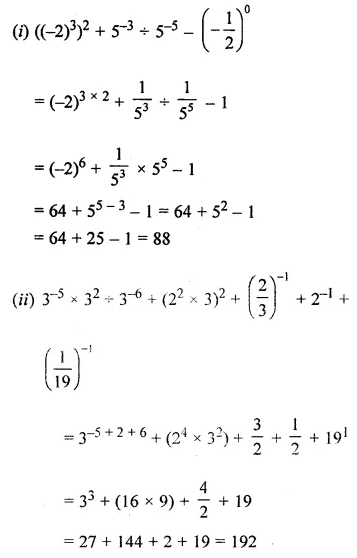Question 8.
Simplify and write in exponential form with negative exponent:Solution: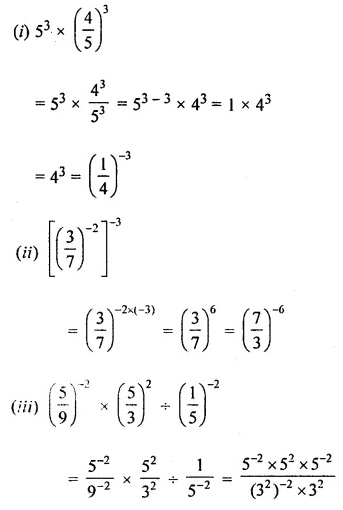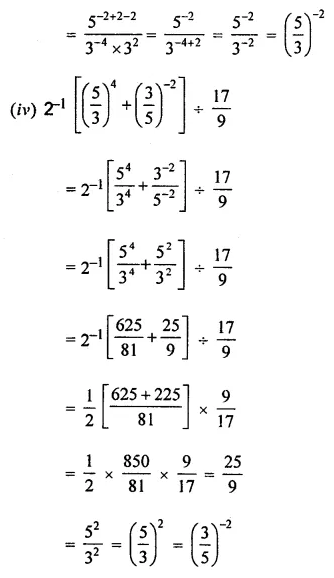Question 9.
Simplify: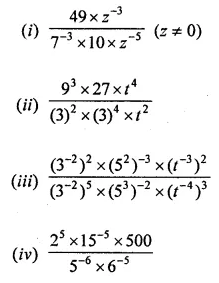Solution: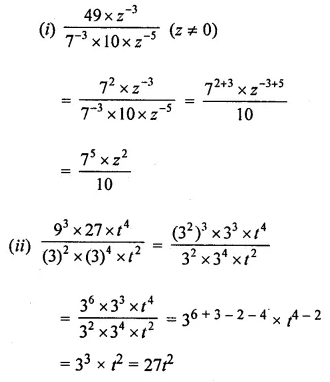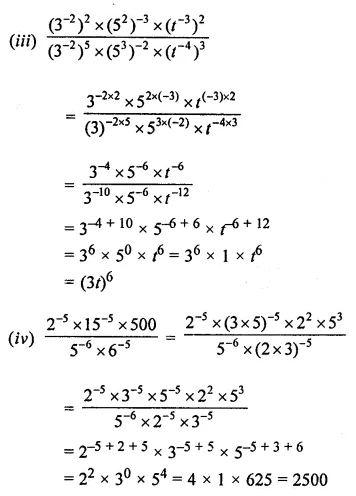Question 10.
By what number should $$\left( \frac { 3 }{ -2 } \right) ^{ -3 }$$ be divided to get $$\left( \frac { 2 }{ 3 } \right) ^{ 2 }$$ ?
Solution:Question 11.
Find the value of m for which 9m ÷ 3-2 = 94.
Solution: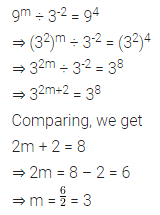Question 12.Solution:Question 13.Solution: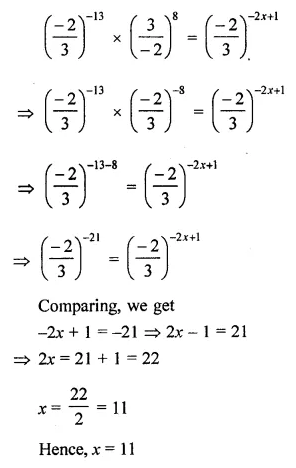Question 14.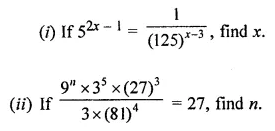Solution: